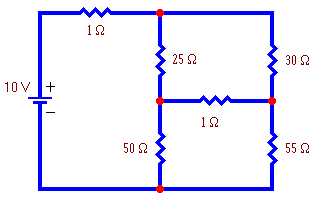# Electronics: Linear AlgebraOne of the most important applications of linear algebra to electronics is to analyze electronic circuits that cannot be described using the rules for resistors in series or parallel such as the one shown to the right. The goal is to calculate the current flowing in each branch of the circuit or to calculate the voltage at each node of the circuit.

Knowing the branch currents, the nodal voltages can easily be calculated, and knowing the nodal voltages, the branch currents can easily be calculated. Loop analysis finds the currents directly and nodal analysis find the voltages directly. Which method is simpler depends on the given circuit. Nodal analysis is important because its answers can be directly compared with voltage measurements taken in a circuit, whereas currents are not so easily measured in a circuit (one must cut wires). Click on one of the following topics:

You may also wish to review the following background material:Written by Eric Hiob, December 31, 1996